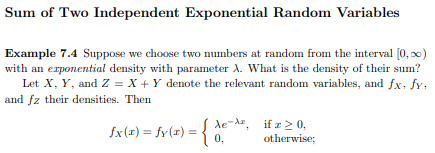# Probability

## Page 303 Q 11

A company buys 100 lightbulbs, each of which has an exponential lifetime of 1000 hours. What is the expected time for the first of these bulbs to burn out? (See Exercise 10.)

$\frac{\mu}{n} = \frac{1000}{100} = 10 hrs$

## Page 303 Q 14

Assume that X1 and X2 are independent random variables, each having an exponential density with parameter λ. Show that Z = X1 − X2 has density $f_Z(z) = (1/2)\lambda e^{−\lambda|z|}$

### Sum of Two Independent Exponential Random VariablesIf x greater than or equal to 0:

$f_X(x) = f_Y(x) = \lambda e^{\lambda x}$ If z > 0:

$f_Z(z) = \int^\infty_{-\infty} f_X(z+y)f_Y(y)dy$ $f_Z(z) = \int^z_0 \lambda e ^{-\lambda (z + y)}\lambda e^ {-\lambda y}dy$

$f_Z(z) = \int^z_0 \lambda ^2 e ^{-\lambda (z+2y)}dy$

$\frac{\lambda}{2} z e^{-\lambda |z|}$

## Page 320 Q 1

Let X be a continuous random variable with mean µ = 10 and variance σ^2 = 100/3. Using Chebyshev’s Inequality, find an upper bound for the following probabilities.

### Chebyshev Inequality

Let X be a discrete random variable with expected value μ = E(X), and let $\sigma > 0$ be any positive real number. Then $P(|X-\mu| \geq \sigma) \leq \frac{V(X)}{\sigma^2}$

cheby <- function(mean, variance, difference){
upper_bound = variance / difference^2
if (variance > difference^2){
return(cat("The upper bound of P(|X -", mean,"| >=",difference,") is", upper_bound, "- insignificant result"))
}
else{
return(cat("The upper bound of P(|X -", mean,"| >=",difference,") is", upper_bound))
}

}

### Part A

1. P(|X − 10| ≥ 2).
mean = 10
var = 100/3
diff = 2
cheby(mean, var, diff)
## The upper bound of P(|X - 10 | >= 2 ) is 8.333333 - insignificant result

### Part B

1. P(|X − 10| ≥ 5).
var = 100/3
diff = 5
cheby(mean, var, diff)
## The upper bound of P(|X - 10 | >= 5 ) is 1.333333 - insignificant result

### Part C

1. P(|X − 10| ≥ 9).
var = 100/3
diff = 9
cheby(mean, var, diff)
## The upper bound of P(|X - 10 | >= 9 ) is 0.4115226

### Part D

1. P(|X − 10| ≥ 20).
var = 100/3
diff = 20
cheby(mean, var, diff)
## The upper bound of P(|X - 10 | >= 20 ) is 0.08333333